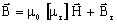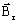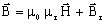# Magnet (vector): linear approximation

## Presentation

These models ( Linear magnet described by the Cartesian / cylindrical / spherical vector Br ) define a B(H) dependence of linear demagnetization in Flux (not available in material manager).

Main characteristics:

• the mathematical model and the direction of magnetization are associated
• same number of materials and/or coordinate systems than regions

## Mathematical model

In the direction of magnetization the model is a straight line.

The corresponding mathematical formula is written as follows:

Β(Η)=μ0μrΗ+ Br

where:

• μ0 is the permeability of vacuum, μ0 = 4 π 10-7 H/m
• μr is the relative permeability (recoil line slope)
• Br is the remanent flux density T

The shape of the B(H) dependence is given in the opposite figure:## Direction of magnetization

The direction of magnetization is “associated” with the model. Three models are provided: Cartesian, cylindrical or spherical vector.

## Cartesian magnetization

The mathematical model is the linear model previously described.

The B(H) dependence is defined by the following relationship in a Cartesian coordinate system:where:

•is the remanent flux density defined by three components following the three axes of the Cartesian coordinate system: ( Brx, Bry, Brz)
• μr is the tensor of relative permeability; the three numerical values ( μrx, μry, μrz) correspond to the three axes of the Cartesian coordinate system## Cylindrical magnetization

The mathematical model is the linear model previously described.

The B(H) dependence is defined by the following relationship in a cylindrical coordinate system:where:

•is the remanent flux density defined by three components following the three axes of the cylindrical coordinate system: ( Brr, B and Brz)
• μ r is the relative permeabilityradial (Brr ) orthoradial (Brθ) axial (Brz)

## Spherical magnetization

The mathematical model is the linear model previously described.

The B(H) dependence is defined by the following relationship in a spherical coordinate system:where:

•is the remanent flux density defined by three components following the three axes of the spherical coordinate system: ( Brr, B et B)
• μ r is the relative permeability

radial (Brr ):